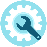﻿ Facit 1113Details:

This electronic calculator was produced (and I guess also developed) by Sharp.  It contains a single Hitachi integrated circuit and a VFD display.  The position of the  decimal point is determined automatically.  The number of digits can be set to 0, 2, 4 or max.   If desired a memory totals all calculation results.

The sign of the calculated result is shown in the utmost right of the display.  At that same position an overflow is shown by a “C” or “E” while a dot at that position indicates that the memory contains a number.

Usage:

Enter a number, then pushing  “ += “ adds the number to the result register which is shown in the display.

- =“ subtracts in the same way.

Multiplication:

Enter the 1st number, push the “X” key, enter the 2nd number, push the “+=” or the  “- =“  key.

Division:

Similar to multiplication using the “:” key.

Usage of the % key:

To add a percentage to a number, enter the number, then multiply it with the percentage using the “X” key, then press the “%” key. The display shows the calculated percentage.  Pushing the “+=” key adds the increase to the number. The display shows the end result.

Example:  22  x  7 %  +=  23.54

Function of some keys, switches etc.

• The upper switches determine special operations:
• :      automatically sums results of calculations  in memory
• K:      uses the displayed number as first operand
• 5/4:   rounds as usual
• 0 | 2 | 4 :  determines the number of digits behind the decimal point
• C”  clears the machine except memory
• CE”  clears last entry
• RM/CM”  reads memory to input and clears memoryClick to view a full size picture (opens in a new tab)

Serial number:

Build in:

Functions:

Power:

Market-introduction:

Production until:

Price:

617 0656

Probably early 1976

Addition, Subtraction, Multiplication & Division

220V

Ca. 1975

Ca. 1976

Probably approx. DM. 250.
In 1976 mechanical machines are history.  At the same time prices of electronic machines are rapidly changing, probably by the increased availability of integrated circuits. That is why prices often aren’t mentioned in publications like in the German Büromaschinen Lexikon.